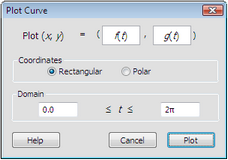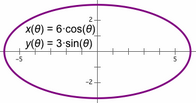# Plot Parametric CurveThis Graph menu command constructs the parametric plot defined by two functions. (If your sketch doesn't contain two functions, a warning dialog box appears.)If one or two functions are selected when you choose this command, the selected functions appear in the edit boxes at the top of the dialog box. The first function is used for x (or r), and the second for y (or θ).

To enter or change the function in either edit box, highlight that edit box and then click the desired function in the sketch. (If the dialog box hides the function you want to click, move the dialog box out of the way.)

Coordinates: Use these radio buttons to choose whether the curve is plotted using Rectangular (x, y) or Polar (r, θ) coordinates.

Domain: Use these edit boxes to determine the domain of the independent variable used to evaluate the functions. You can type a number or an expression in either box.Once you’ve constructed a parametric plot, use the Plot Properties dialog box to change the domain, the number of samples, or the display of arrowheads at the ends of the curve. (Drag these arrowheads as another way to change the domain.)